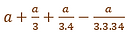top of page
##### Value of Root 3 and Root 2

Now, the question arises how the values of √3 was derived by Shulbakaras. The authors of Shulba were aware of irrational numbers. We find the instances of the application to cases of rectangles whose sides cannot be represented by rational quantities. For instance, Sautramaniki vedi, the application is made of a right-angled triangle (15/√3, 36/√3, 39/√3)   and Ashvamedhiki vedi of the right-angled triangle  15/√2  36/√2, 39/√2 .

#### Value of √2

The most important achievement of sulbasutra geometry is the enunciation of a theorem of the square on the diagonal and the recognition of properties of similar figures. The doubling of square i.e., the geometrical construction of   is necessary for the construction of one of three primarily essential altars of the Vedic sacrifices viz., the Dakshina. In the connection with the construction of the Rathachakra citi, as in the case of most Kamya citi, one has to first draw a square equal to the primitive and standard agni, the chaturasra syanciti whose form consists partly of squares and partly of rectangles It is clear that the solution to this problem is possible without the help of theorem of the square of the diagonal. For Prauga citi, again one has to double the square. There are many instances available in sulbasutras where the applications of a theorem of the square of the diagonal are mentioned for the construction of vedis. Shulbakaras pointed out a case of geometrical construction in which the number expressing the square of the diagonal of a square is closer to a square number.

Baudhayana Shulbasutra states,

समस्य द्विकरणि प्रमाणं तृतीयान वर्धयेत् तच्चतुर्थेनात्मचतुस्त्रिंशोनेन|| १.६१||

“Increase the measure of the square by its third and this third by its fourth less the thirty-fourth part of this fourth”.

This rule gives the ratio of the diagonal of the squares to the side of a square. The value of the diagonal(savishesha) can be expressed as                           where a is the side of the given square.  This is the formula for finding out the measure of the diagonal of a square. There is a definite relationship between the side and diagonal of the square. That is the area of a square described on the diagonal of a square is double the area of that square rule (1.45 Baudhayana shulbasutra) .

d  = 2a  where d is the diagonal and a is the side of square.

d/a = √2,  …..(1)

As per the rule 1.61 of Baudhayana Shulbasutra d(savishesha of a) =                                …….(2)

From (1) and (2)   √2 = d/a

It means ,

This is the numerical formula to express the relation of the diagonal of a square to the side of a square. In decimal fractions, the value comes out to 1.4142156 … which is correct up to five decimal place.

Geogebra computational  Tool for finding value of √2

2

2#### Value of √3

According to Katyayana Shulbasutra, to construct the Ashvamedha fire altar √3 is used.  The area of the Ashvamadha altar should be either double or triple that of the basic fire altar.  In case, if the area of Ashvamedha is triple that of the basic altar then the role of √3 becomes important. The area of the basic fire altar is√(15/2) thus sides of the Ashvamedha fire altar will be√(15/2)*(√(3) = √(45/2).The area of Sautramani Vedi should be one-third that of Mahavedi (area = 972 square prakramas- measuring east side 24, west side 30, east-west line,36 prakramas) then, the area of Sautramani Vedi  is 324 prakramas measuring  8√3, 10√3,12√3. The authors of sulbasutras also followed the case in which the number expressing the required square having an area three times that of a given square is closer to a square number.

Katyayana Shulbasutra mentions method of finding the value of √3 as,

प्रमाण तिर्यक् द्विकरण्यायामस्तस्याक्ष्णया रज्जुस्त्रिकरणी || कात्यायनशुल्बसुत्रम्||२.१४||

“Take the pramana(measure equal to the praci of a given square) as tiryanmani and dvikarani(diagonal of the given square) as the ayama (length) of the required oblong, then the aksnyarajju(diagonal) of such an oblong is called trikarani (the producer of an area equal to three times that of the given square(K.S 2.14)”.Let ABCD be a given square such that AB = 1 and AC =  , Draw ACEF such that AF = CE = AB = 1 Then, AE is the trikaranai (√3) and will produce a square AEGH having the area equal to three times that of ABCD      AE2 =   AC2 + EC2. (Baudhayana 1.48)

= (√2)^2+(1)^2.

= 3

AE = √3      This rule is based upon the Baudhayana theorem.

Finding the value of √3

Katyayana sulbasutra mentions the case of finding the value of  as 26/15.

Take square ABCD measuring 15*15 units and two other squares of similar size and divide each into 15*15 = 225 small squares. Combine all the squares into one.  The resultant figure will have 675 small squares and is very nearly to a complete square(26*26 = 676)

Now, add one small square in the south- east corner to complete the square. The square AB’C’D’ thus obtained, be very nearly to the required square. The line AB’ will represent trikarani(√3)

AB’ = AB +BE + EB’

√3

Computational tool for finding value of √3

bottom of page